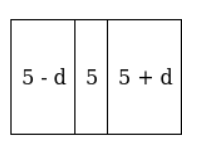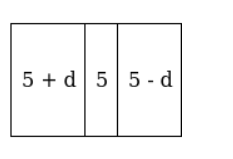# The digits of a 3 - digit number are in AP, and their sum is 15.

Question:

The digits of a 3 - digit number are in AP, and their sum is 15. The number obtained by reversing the digits is 594 less than the original number. Find the number.

Solution:

To Find: The number

Given: The digits of a 3 – digit number are in AP, and their sum is 15.

Let required digit of 3 - digit number be (a - d), (a), (a + d). Then,

$(a-d)+(a)+(a+d)=15 \Rightarrow 3 a=15 \Rightarrow a=5$

(Figure show 3 digit number original number)(Figure show 3 digit number in reversing form)So, $(5+d) \times 100+5 \times 10+(5-d) \times 1=\{(5-d) \times 100+5 \times 10+(5+d) \times 1\}-594$

$200 d-2 d=-594 \Rightarrow d=-3$ and $a=5$

So the original number is 852